# Class 12 Maths NCERT Solutions for Chapter 13 Probability Exercise 13.5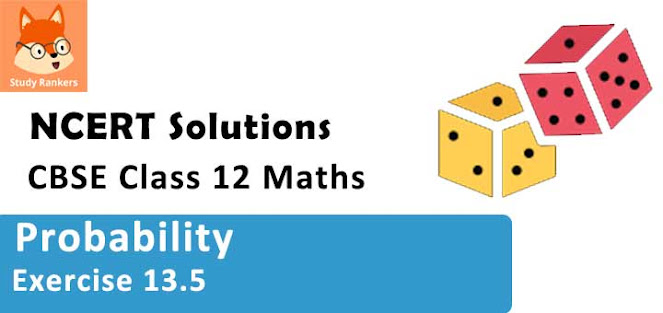### Probability Exercise 13.5 Solutions

1. A die is thrown 6 times. If ‘getting an odd number’ is a success, what is the probability of
(i) 5 successes?
(ii) at least 5 successes?
(iii) at most 5 successes?

Solution

The repeated tosses of a die are Bernoulli trials. Let X denote the number of successes of getting odd numbers in an experiment of 6 trials.
Probability of getting an odd number in a single throw of a die is, p = 3/6 = 1/2
∴ q = 1 – p = 1/2
X has a binomial distribution.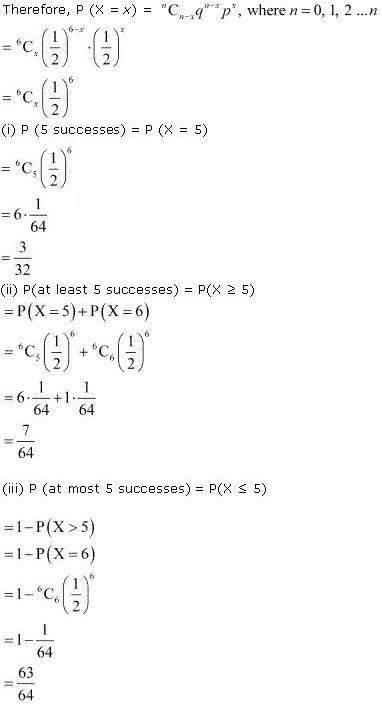2. A pair of dice is thrown 4 times. If getting a doublet is considered a success, find the probability of two successes.

Solution

The repeated tosses of a pair of dice are Bernoulli trials. Let X denote the number of times of getting doublets in an experiment of throwing two dice simultaneously four times.
Probability of getting doublets in a single throw of the pair of dice is
P = 6/36 = 1/6
∴ q = 1 - p = 1 - 1/6 = 5/6
Clearly, X has the binomial distribution with n = 4, P = 1/6, and q = 5/6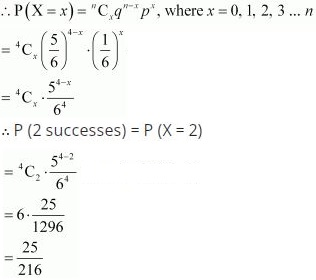3. There are 5% defective items in a large bulk of items. What is the probability that a sample of 10 items will include not more than one defective item?

Solution

Let X denote the number of defective items in a sample of 10 items drawn successively. Since the drawing is done with replacement, the trials are Bernoulli trials.
⇒ P = 5/100 = 1/20
∴ q = 1 - 1/20 = 19/20
X has a binomial distribution with n = 10 and p = 1/20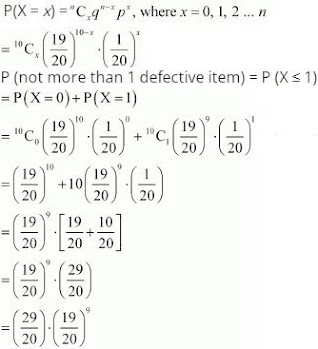4. Five cards are drawn successively with replacement from a well-shuffled deck of 52 cards. What is the probability that
(i) all the five cards are spades?
(ii) only 3 cards are spades?

Solution

Let X represent the number of spade cards among the five cards drawn. Since the drawing of card is with replacement, the trials are Bernoulli trials.
In a well shuffled deck of 52 cards, there are 13 spade cards.
⇒ P = 13/52 = 1/4
∴ q = 1 - 1/4 = 3/4
X has a binomial distribution with n = 5 and p = 1/4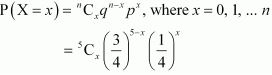(i) P (all give cards are spades) = P(X = 5)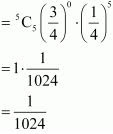(ii) P(only 3 cards are spades) = P(X = 3)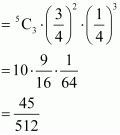(iii) P (none is a spade) = P(x = 0)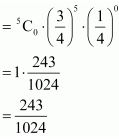5. The probability that a bulb produced by a factory will fuse after 150 days of use is 0.05. What is the probability that out of 5 such bulbs
(i) none
(ii) not more than one
(iii) more than one
(iv) at least one
will fuse after 150 days of use.

Solution

Let X represent the number of bulbs that will fuse after 150 days of use in an experiment of 5 trials. The trials are Bernoulli trials.
It is given that, p = 0.05
∴ q = 1 - p = 1 - 0.05 = 0.95
X has a binomial distribution with n = 5 and p = 0.05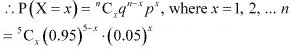(i) P (none) = P(x = 0)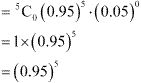(ii) P(not more than one) = P(X ≤ 1)
= P(X = 0) + P(x = 1)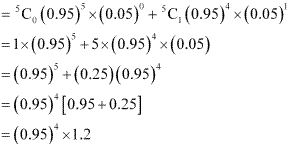(iii) P (more than 1) = P(X > 1)
= 1 - P(X ≤ 1)
= 1 - P(not more than 1)
= 1 - (0.95)4 × 1.2
(iv) P (at least one) = P(X ≥ 1)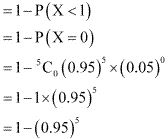6. A bag consists of 10 balls each marked with one of the digits 0 to 9. If four balls are drawn successively with replacement from the bag, what is the probability that none is marked with the digit 0?

Solution

Let X denote the number of balls marked with the digit 0 among the 4 balls drawn.
Since the balls are drawn with replacement, the trials are Bernoulli trials.
X has a binomial distribution with n = 4 and p = 1/10
∴ q = 1 - p = 1 - 1/10 = 9/10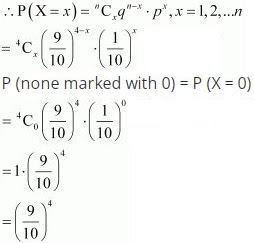7. In an examination, 20 questions of true-false type are asked. Suppose a student tosses a fair coin to determine his answer to each question. If the coin falls heads, he answers ‘true’; if it falls tails, he answers ‘false’. Find the probability that he answers at least 12 questions correctly.

Solution

Let X represent the number of correctly answered questions out of 20 questions.
The repeated tosses of a coin are Bernoulli trails. Since “head” on a coin represents the true answer and “tail” represents the false answer, the correctly answered questions are Bernoulli trials.
∴ p = 1/2
∴ q = 1 - p = 1 - 1/2 = 1/2
X has a binomial distribution with n = 20 and p = 1/2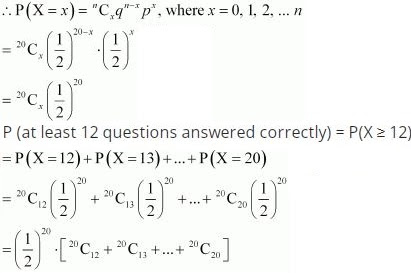8. Suppose X has a binomial distribution B(6,1/2). Show that X = 3 is the most likely outcome.
(Hint: P(X = 3) is the maximum among all P (xi), xi = 0, 1, 2, 3, 4, 5, 6)

Solution

X is the random variable whose binomial distribution is B (6, 1/2).
Therefore, n = 6 and p = 1/2
∴ q = 1 - p = 1 - 1/2 = 1/2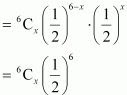It can be seen that P(X = x) will be maximum, if 6Cx will be maximum.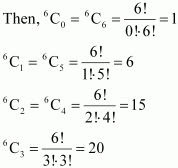The value of  6Cx is maximum. Therefore, for x = 3, P(X = x) is maximum.
Thus, X = 3 is the most likely outcome.

9. On a multiple choice examination with three possible answers for each of the five questions, what is the probability that a candidate would get four or more correct answers just by guessing?

Solution

The repeated guessing of correct answers from multiple choice questions are Bernoulli trials. Let X represent the number of correct answers by guessing in the set of 5 multiple choice questions.
Probability of getting a correct answer is, p = 1/3
∴ q = 1 - p = 1 - 1/3 = 2/3
Clearly, X has a binomial distribution with n = 5 and p = 1/3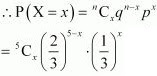P(guessing more than 4 correct answers) = P(X ≥ 4)
= P(X = 4) + P(X = 5)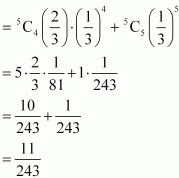10. A person buys a lottery ticket in 50 lotteries, in each of which his chance of winning a prize is 1/100. What is the probability that he will in a prize (a) at least once (b) exactly once (c) at least twice?

Solution

Let X represent the number of winning prizes in 50 lotteries. The trials are Bernoulli trials.
Clearly, X has a binomial distribution with n = 50 and p = 1/100
∴ q = 1 - p = 1 - 1/100 = 99/100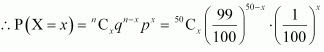(a) P(winning at least once) = P(X ≥ 1)
= 1 - P(X < 1)
= 1 - P(X = 0)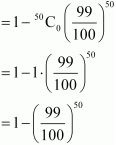(b) P (winning exactly once) = P(X = 1)(c) P (at least twice) = P(X ≥ 2)
= 1 - P(X < 2)
= 1 - P(X ≤ 1)
= 1 - [P(X = 0) + P(X = 1)]
= [1 - P(X = 0)] - P(X = 1)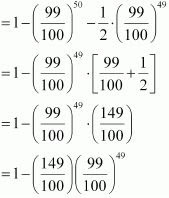11. Find the probability of getting 5 exactly twice in 7 throws of a die.

Solution

The repeated tossing of a die are Bernoulli trials. Let X represent the number of times of getting 5 in 7 throws of the die.
Probability of getting 5 in a single throw of the die, p = 1/6
∴ q = 1 - p = 1 - 1/6 = 5/6
Clearly, X has the probability distribution with n = 7 and p = 1/6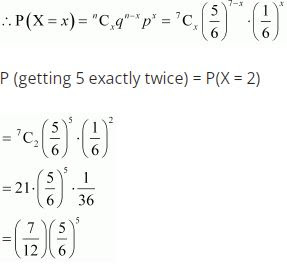12. Find the probability of throwing at most 2 sixes in 6 throws of a single die.

Solution

The repeated tossing of the die are Bernoulli trials. Let X represent the number of times of getting sixes in 6 throws of the die.
Probability of getting six in a single throw of die, p = 1/6
∴ q = 1 - p = 1 - 1/6 = 5/6
Clearly, X has a binomial distribution with n = 6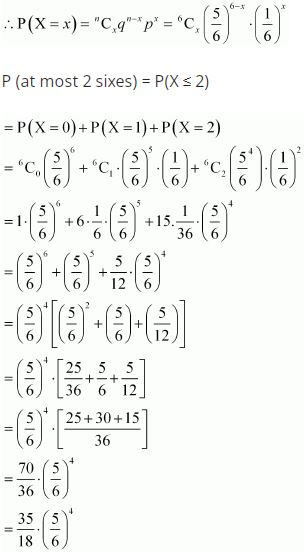13. It is known that 10% of certain articles manufactured are defective. What is the probability that in a random sample of 12 such articles, 9 are defective?

Solution

The repeated selections of articles in a random sample space are Bernoulli trails. Let X denote the number of times of selecting defective articles in a random sample space of 12 articles.
Clearly, X has a binomial distribution with n = 12 and p = 10% = 10/100 = 1/10
∴ q = 1- p = 1 - 1/10 = 9/10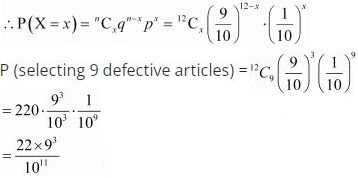14. In a box containing 100 bulbs, 10 are defective. The probability that out of a sample of 5 bulbs, none is defective is
(A) 10-1
(B) (1/2)4
(C) (9/10)5
(D) 9/10

Solution

The repeated selections of defective bulbs from a box are Bernoulli trials. Let X denote the number of defective bulbs out of a sample of 5 bulbs.
Probability of getting a defective bulb, p = 10/100 = 1/10
∴ q = 1 - p = 1 - 1/10 = 9/10
Clearly, X has a binomial distribution with n = 5 and p = 1/10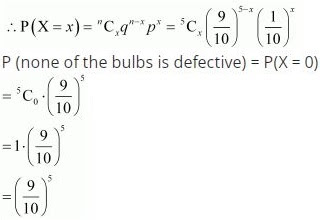15. The probability that a student is not a swimmer is 1/5 . Then the probability that out of five students, four are swimmers is

(A) 5C4 (4/5)4 1/5

(B) (4/5)4 1/5

(C) 5C1 1/5(4/5)4

(D) None of these

Solution

The repeated selection of students who are swimmers are Bernoulli trials. Let X denote the number of students, out of 5 students, who are swimmers.
Probability of students who are not swimmers, q = 1/5
∴ p = 1 - q = 1 - 1/5 = 4/5
Clearly, X has a binomial distribution with n  = 5 and p = 4/5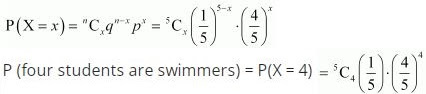Therefore, the correct answer is A.